Banker's algorithm is used for deadlock avoidance.

Prob. Consider the following snapshot of a system-
Answer the following questions using the Banker’s algorithm-

(i)                 What is the content of the matrix need?
(ii)               Is the system in a safe state?

(iii)             If a request from process P1 arrives for (0,4,2,0), can the request be granted immediately?

Ans.

Banker’s algorithm: Need Calculation

Steps to calculate need:

Step 1: in row of process P0, use formula
Need=Max – Allocation

Step 2: Follow step 1 above for all other processes i.e. P1, P2, P3, P4, P5.

Result given below.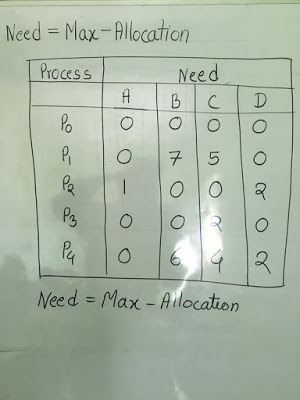Bankers algorithm: Need calculation

Steps to calculate Safe state: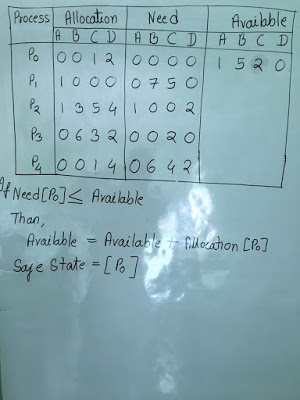Image 1

Step 1: Find the process which have Need lesser than Available.

If need of process is lesser than available, add its allocation to theavailable and remove that process from the table.

Here as shown below,

Need [Process P0]  is less than Available
Than,
New Available = Available + Allocation [Process P0] (Available value is updated in Image 2)
So ,
Safe state = [P0].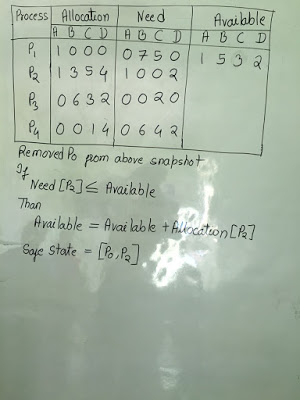Image 2

Step 2: In STEP 1, We find the process P0 which have Need lesser than Available.
We removed Process P0 from table, and updated the Available. (See Image 2).

Now we repeat Step 1 as explain above.

Here as shown below,

Need [Process P2]  is less than Available
Than,
New Available = Available + Allocation [Process P2](Available value is updated in Image 3)
So ,

Safe state =  [P0, P2].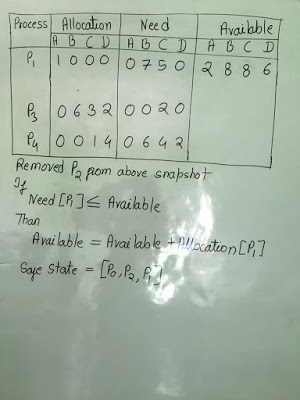Image 3

Step 3: In STEP 2, we find the process P2 which have Need lesser than Available.
We removed Process P2 from table, and updated the Available.(See Image 3).

Now we repeat Step 1 as explain above.

Here as shown below,

Need [Process P1]  is less than Available
Than,
New Available = Available + Allocation [Process P1](Available value is updated in Image 4)
So ,

Safe state = [P0, P2, P1].Image 4

Step 4: In STEP 3, we find the process P1 which have Need lesser than Available.
We removed Process P1 from table, and updated the Available.(See Image 4).

Now we repeat Step 1 as explain above.

Here as shown below,

Need [Process P3]  is less than Available
Than,
New Available = Available + Allocation [Process P3](Available value is updated in Image 5)
So ,

Safe state = [P0, P2, P1, P3].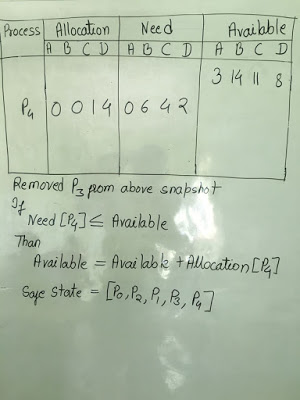Image 5

Step 5: In STEP 4, we find the process P3 which have Need lesser than Available.
We removed Process P3 from table, and updated the Available.(See Image 5).

Now we repeat Step 1 as explain above.

Here as shown below,

Need [Process P4]  is less than Available

So ,

Safe state = [P0, P2, P1, P3, P4].

As all the processes comes under safe state, so system is in a safe state.